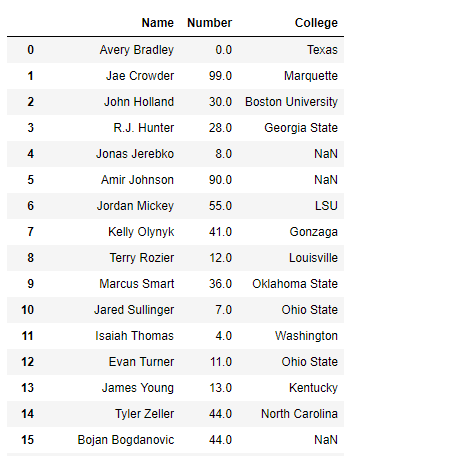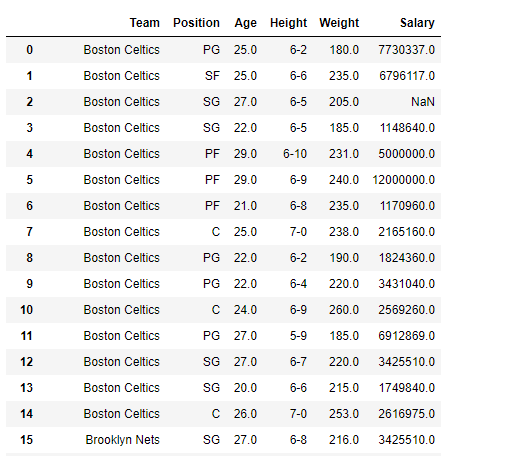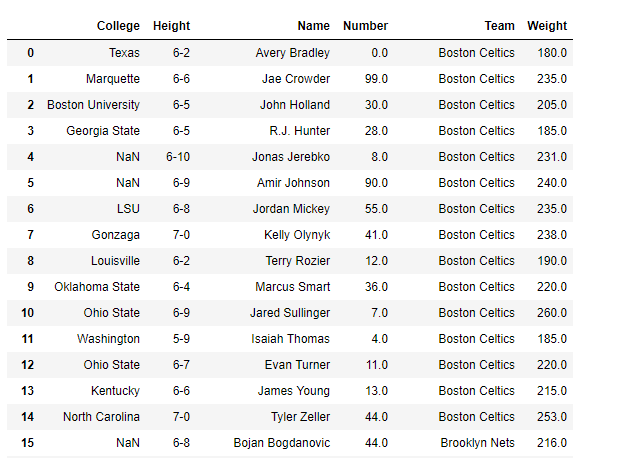Open in App
Not now

# Creating views on Pandas DataFrame | Set – 2

• Last Updated : 26 Jan, 2019

Prerequisite: Creating views on Pandas DataFrame | Set – 1

Many times while doing data analysis we are dealing with a large data set has a lot of attributes. All the attributes are not necessarily equally important. As a result, we want to work with only a set of columns in the dataframe. For that purpose, let’s see how we can create views on the Dataframe and select only those columns that we need and leave the rest.

Given a Dataframe containing nba data, create views on it such that only desired columns are included.

Solution #1: While reading the data from the csv file into Python, We can select all those columns that we want to read into the DataFrame.

 `# importing pandas as pd``import` `pandas as pd`` ` `# list of columns that we want to``# read into the DataFrame``use_cols ``=``[``'Name'``, ``'Number'``, ``'College'``]`` ` `# Reading the csv file``df ``=` `pd.read_csv(``'nba.csv'``, usecols ``=` `lambda` `x : x ``in` `use_cols,``                                             ``index_col ``=` `False``)`` ` `# Print the dataframe``print``(df)`

Output :Solution #2 : While reading the data from the csv file into Python, we can list all those columns that we do not want to read into the DataFrame. It is like dropping those columns.

 `# importing pandas as pd``import` `pandas as pd`` ` `# list of columns that we do not want``# to read into the DataFrame``skip_cols ``=``[``'Name'``, ``'Number'``, ``'College'``]`` ` `# Reading the csv file``df ``=` `pd.read_csv(``'nba.csv'``, usecols ``=` `lambda` `x : x ``not` `in` `skip_cols,``                                                  ``index_col ``=` `False``)`` ` `# Print the dataframe``print``(df)`

Output :Solution #3 : We can use the `difference()` method to drop the columns that we do not need.

 `# importing pandas as pd``import` `pandas as pd`` ` `# Reading the csv file``df ``=` `pd.read_csv(``"nba.csv"``)`` ` `# Print the dataframe``print``(df)`

Output :Now we will drop those columns which we do not need by using the `difference()` method.

 `# Drop the listed columns``df_view ``=` `df[df.columns.difference([``'Position'``, ``'Age'``, ``'Salary'``])]`` ` `# Print the new DataFrame``print``(df_view)`

Output :My Personal Notes arrow_drop_up